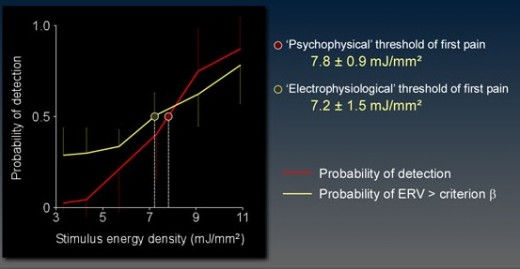top of page

# Single-trial analysis of ERPs

As shown in the following figure, single-trial EEG signal amplitude may be correlated with perceptual measures such as intensity of perception, thereby giving access to 'single-trial brain dynamics'.ERP images

An interesting way of visualizing ERP data has been proposed by Makeig et al. A colored image is plotted using a collection of single-trial data epochs, optionally sorted on and/or aligned to an input sorting variable and smoothed across trials with a moving-average.Single-trial detection of event-related brain potentials using the wavelet transform of EEG epochs

It was assumed that decomposing epochs in both time and frequency would allow disentangling of stimulus-locked and frequency-steady EEG changes from background EEG and non-cerebral artefacts. As further described, the TF filter used to enhance single-trial EEG responses was electrophysiologically determined in a preliminary recording session. The method yielded, for each single EEG epoch, an ‘EEG response value’ (ERV) hypothesized to predict the presence or absence of electrophysiological correlates of nociceptor activation. This hypothesis was tested in a second recording session.

Recordings of the preliminary session were used to produce a weighted TF filter (W) identifying the TF distribution of EEG changes induced by nociceptor activation. To produce this TF filter, only supra-threshold stimulus intensity trials were used. The filter was computed by averaging the amplitude estimate (obtained using the Continuous Morlet Transform) of these trials. The result was normalized using, for each frequency row, the average of amplitudes enclosed in the foreperiod between 2400 and 2100 ms. The result expresses the average relative increase or decrease of oscillation amplitude as a function of time and frequency (see Figure: W). As amplitudes were averaged regardless of phase, this procedure enhanced stimulus-related EEG changes both phase-locked (i.e. event-related potentials) and non-phase-locked (i.e. event-related synchronization and desynchronization) to stimulus onset.

Each CMT of the second recording session was normalized. The result expresses, for each single EEG epoch, the relative increase or decrease of oscillation amplitude (see Figure CMTi, one epoch shown as an example). CMTi was then multiplied by the previously computed weighting matrix W (see Figure: W·CMTi). This operation can be considered as applying a TF filter to the single-trial data. Finally, the sum of TF amplitude values ranging from 0 to 700 ms was calculated. It was hypothesized that this ERV would allow identification of the presence or absence of electrophysiological correlates of nociceptor activation.The time-frequency matrix (W) can be considered as an electrophysiologically determined weighted time-frequency filter. Near-zero weights correspond to time-frequency localities where the studied event did not induce important changes in EEG amplitude while positive or negative weights correspond to time-frequency localities where the studied event induced either an increase or a decrease of oscillation amplitude. Applying this time-frequency filter to single EEG epochs should thus allow filtering of EEG fluctuations not occurring at the latency and frequency of event-related responses.A Receiver operating characteristic (ROC) curve assessing the discriminatory performance of the ERV was computed for each single EEG epoch. RT was used to define the state of each trial. Detected trials were considered as trials where detection was mediated by nociceptor activation, were compared to undetected trials. The area under the curve, an index of discriminatory performance, was 0.800 +/- 0.023 and significantly (P < 0.0001) greater than 0.5 (no predictive value). A decision criterion (b) was chosen such that specificity (0.76 +/- 0.11) equalled sensitivity (0.74 +/- 0.09). Probability of detection curves as a function of stimulus intensity (six different intensities ranging from 3.4 to 10.9 mJ/mm2). The absolute probability of detection is plotted as a dashed line. The probability of detecting the stimulus is plotted as a red line. The probability that ERV exceeded the decision criterion (b) is plotted as a yellow line. Error bars quantify the standard deviation across subjects. Curves were used to estimate the the psychophysical threshold of first pain (7.8 +/- 0.9 mJ/mm2) and the electrophysiological threshold of nociceptor-related EEG responses (7.2 +/- 1.5 mJ/mm2) for P=0.5.

bottom of page